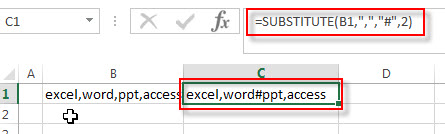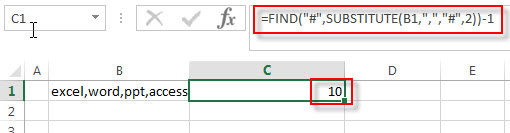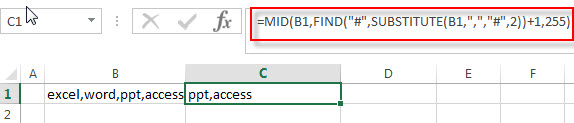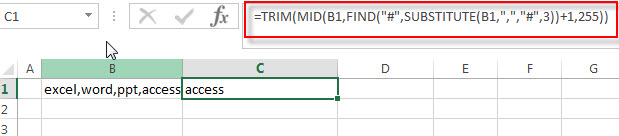# How to extract text after the second or nth specific character (space or comma)

In the previous post, we talked that how to extract text after the first occurrence of the comma character in excel. And this post explains that how to get a substring after the second or nth occurrence of the comma or space character in excel.

## Extract text after the second or nth specific character

If you want to extract text after the second or nth comma character in a text string in Cell B1, you need firstly to get the position of the second or nth occurrence of the comma character in text, so you can use the SUBSTITUTE function to replace the second comma character with the hash character, then using the FIND function to look for the first hash character in text returned by substitute function, the returned value is the position of the second occurrence of the comma character in text. Last, you can use the MID function to extract a substring that you want to extract.

So you can create a formula based on the MID function, the FIND function and the SUBSTITUTE function as follows:

`=TRIM(MID(B1,FIND("#",SUBSTITUTE(B1,",","#",2))+1,255))`

Let’s see how this formula works:

=SUBSTITUTE(B1,”,”,”#”,2)This function replaces the second comma character with hash character in cell B1, and the returned result goes into the FIND function as its within_text argument.

=FIND(“#”,SUBSTITUTE(B1,”,”,”#”,2))+1The FIND function will return the position of the first occurrence of the hash character in within_text string returned by the SUBSTITUTE function. Then add 1 to get the starting position after the second occurrence of the comma character in text. And it returns 11. It is fed into the MID function as its start_num argument.

=MID(B1,FIND(“#”,SUBSTITUTE(B1,”,”,”#”,2))+1,255)The MID function will extracts 255 characters from a text string in Cell B1, starting at the 11character.

=TRIM()

The TRIM function removes all extra space characters and just leave only one between words.

If you want to extract a substring after the third occurrence of the comma character, you can use the following formula:

=TRIM(MID(B1,FIND(“#”,SUBSTITUTE(B1,”,”,”#”,3))+1,255))### Related Formulas

• Extract Text between Parentheses
If you want to extract text between parentheses in a cell, then you can use the search function within the MID function to create a new excel formula…
• Extract Text between Brackets
If you want to extract text between brackets in a cell, you need to create a formula based on the SEARCH function and the MID function….
• Extract Text between Commas
To extract text between commas in Cell B1, you can use the following formula based on the SUBSTITUTE function, the MID function and the REPT function…..
• Extract word that starting with a specific character
Assuming that you have a text string that contains email address in Cell B1, and if you want to extract word that begins with a specific character “@” sign, you can use a combination with the TRIM function, the LEFT function, the SUBSTITUTE function ….
• Extract text before first comma or space
If you want to extract text before the first comma or space character in cell B1, you can use a combination of the LEFT function and FIND function….
• Extract text after first comma or space
If you want to get substring after the first comma character from a text string in Cell B1, then you can create a formula based on the MID function and FIND function or SEARCH function ….
• Extract text before the second or nth specific character
you can create a formula based on the LEFT function, the FIND function and the SUBSTITUTE function to Extract text before the second or nth specific character…

### Related Functions

• Excel MID function
The Excel MID function returns a substring from a text string at the position that you specify.The syntax of the MID function is as below:= MID (text, start_num, num_chars)….
• Excel FIND function
The Excel FIND function returns the position of the first text string (sub string) within another text string.The syntax of the FIND function is as below:= FIND(find_text, within_text,[start_num])…
• Excel SEARCH function
The Excel SEARCH function returns the number of the starting location of a substring in a text string.The syntax of the SEARCH function is as below:= SEARCH  (find_text, within_text,[start_num])…
• Excel Substitute function
The Excel SUBSTITUTE function replaces a new text string for an old text string in a text string.The syntax of the SUBSTITUTE function is as below:= SUBSTITUTE  (text, old_text, new_text,[instance_num])….
• Excel TRIM function
The Excel TRIM function removes all spaces from text string except for single spaces between words.  You can use the TRIM function to remove extra spaces between words in a string.The syntax of the TRIM function is as below:= TRIM (text)….

Sidebar